# Question:

How do I use Remap Constraint?

# Applies to:

• Remap Contsraint
• Topology Optimization

The Remap Constraint block can generate novel geometry with Topology Optimization in nTop. It enforces geometry-based constraints on the design space by leveraging nTop’s unique ability to manipulate scalar and vector fields. The block is named after the Remap Field block that follows a similar technique to perform geometric operations on implicit bodies like shape transformation, mirroring, sectioning, patterning, surface extrudes and many more applications. Here’s an example of a weave pattern generated from cylindrical wires with the Remap Field block.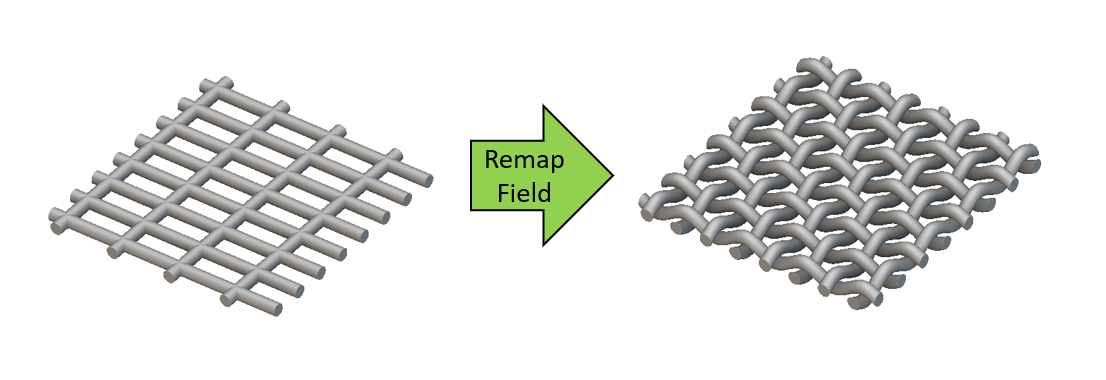While the Remap Field block can be used to create geometric projections by manipulating scalar fields from implicit bodies, the Remap Constraint block can utilize these projections to constrain the density field of the design volume in an optimization problem. This enables users to impose geometry-based constraints such as extrusion, pattern repetition and symmetry, combine these constraints or invent a novel constraint that results in an optimized geometry unique to nTop! We recommend that you learn a little about signed distance fields, the inputs of the Remap Field and the mechanism behind the geometric projections it enables to use the Remap Constraint effectively. This knowledge base video and a learning center course covers these topics in great detail, which will be summarized in the next few paragraphs.

## Remap primer

All implicit bodies in nTop are represented as fields called ‘signed distance fields.’ These fields are generated by signed distance functions which are equations that usually represent geometry . For instance the signed distance function of a sphere of radius ‘r’ at the origin of an orthogonal space is :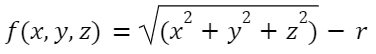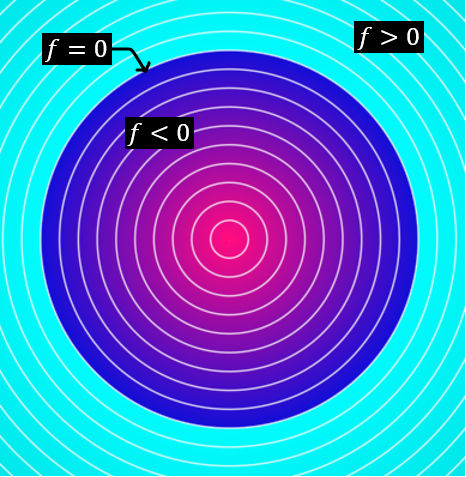This function evaluates to a negative value at every point in space within the sphere and a positive value at every point outside. The sphere's surface, where the function is 0, is the implicit representation of the sphere. To perform a geometric operation on this sphere, you can manipulate the x, y, z components of this function using the Remap Field block. For instance, to stretch the sphere in the x direction by twice its original length, we can substitute the ‘x’ term in the Remap Field input by ‘x/2’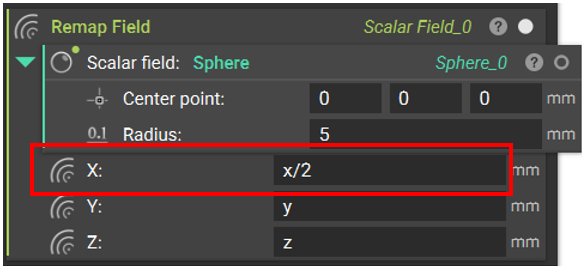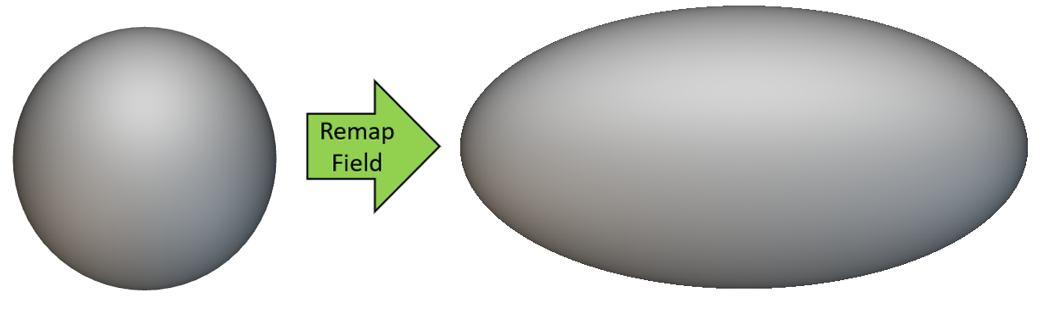This essentially modifies the field defined by equation f  to g to generate an implicit ellipsoid :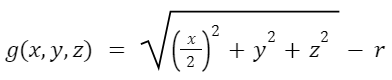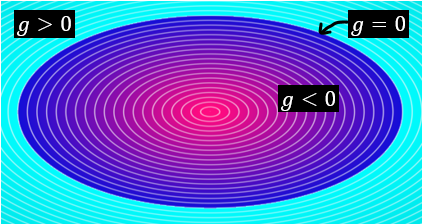Besides implicit bodies, visualizing the field generated by each component (x, y and z) can be used to predict the final state of the implicit body when an operation is performed on a component. In the case of the sphere-to-ellipsoid transformation, the field generated by the x component is stretched (by two times its original value) with the x/2 input in the Remap Field block. Let’s take another example where the x component of the Remap Field input is modified with an Abs() block. The Abs() block outputs the absolute value of a numerical input. Hence the x component will turn into a symmetric signed distance field with positive values on either side of the yz-plane (x=0), as illustrated by the following images.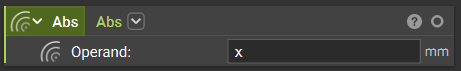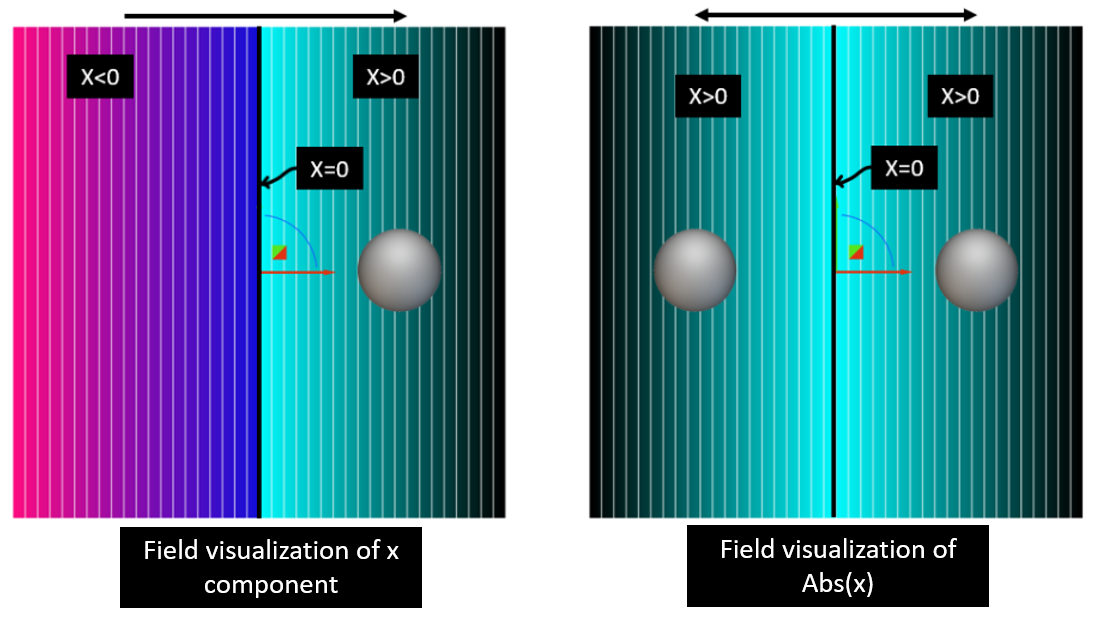As a result of this field modification, any object created on the right-side of the yz-plane will be mirrored to its left side, like the sphere shown in the image above. This concept can be extended to Topology Optimization using the Remap Constraint. We can use the Abs function with any orthogonal components to impose symmetry to the density field generated by the Topology Optimization.

# Examples:

#### 1. Remap Constraint for Symmetry

Here is an example of a Topology Optimization with symmetry about the yz-plane (x component) and the xy-plane (z component) enforced by a remap constraint. The equation gives the input to the remap block:

`g(x,y,z) = (Abs(x),y,Abs(z))`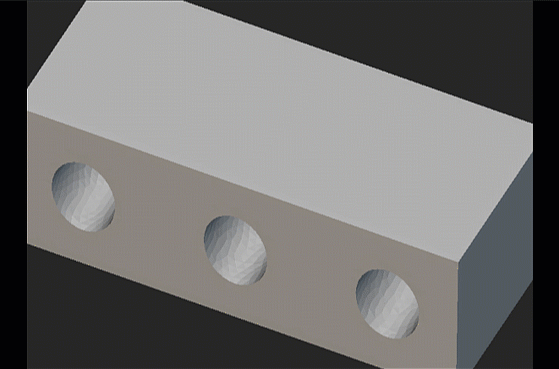Besides the Remap Constraint block, this Topology Optimization setup has the following features that yields the final optimized volume :

• A minimize Structural Compliance objective for a set of loads at the holes on the sides with the hole at the center completely restrained.
• A Voume Fraction Constraint that keeps the volume of the optimized geometry under 25% of the original design volume.
• A Passive Region Constraint that prevents material removal from the hole at the center.

Example file